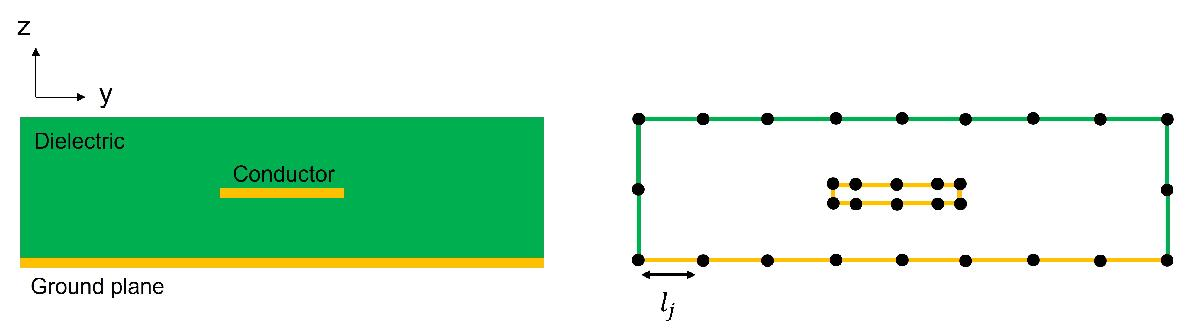## 2-D Field Solver

The 2-D field solver in RF PCB Toolbox™ allows you to model and analyze the cross sections of multiconductor transmission lines in a multilayered dielectric above the ground plane such as a microstrip line. For more detailed information, see 

The four primary transmission line parameters are the resistance R, the inductance L, the conductance G and the capacitance C. You can obtain these parameters by applying a total charge on the conductor-to-dielectric interfaces and a polarization charge on the dielectric-to-dielectric interfaces and solving the created electrostatic field created. The 2-D field solver uses pulse approximation for the total charge σT and discretizes the contours of the conductor-to-dielectric and dielectric-to-dielectric interfaces using straight-line segments. The densities of the segments are controlled by the distribution of nodes along the contours. This figure shows the discretization for a single-conductor transmission line in a single-layered dielectric above the ground plane.At any point p in the YZ plane and above the ground plane, the potential ϕ is due to the combination of σT and the image of σT about the ground plane.

`$\varphi \left(p\right)=\frac{1}{2\pi {e}_{0}}\sum _{j=1}^{J}\int {\sigma }_{T}\left({p}^{\text{'}}\right)\left(\frac{|p-{\stackrel{^}{p}}^{\text{'}}|}{|p-{p}^{\text{'}}|}\right)dl\text{'}$`

where lj is the contour of jth interface in the YZ plane, dl' is the differential element of length at p' on lj, and $\stackrel{^}{p}\text{'}$ is the image of p' about the ground plane.

The electric field is then given by φ

`$E\left(p\right)=\frac{1}{2\pi {e}_{0}}\sum _{j=1}^{J}\int {\sigma }_{T}\left({p}^{\text{'}}\right)\left(\frac{p-{p}^{\text{'}}}{{|p-{p}^{\text{'}}|}^{2}}-\frac{p-{\stackrel{^}{p}}^{\text{'}}}{{|p-{\stackrel{^}{p}}^{\text{'}}|}^{2}}\right)d{l}^{\text{'}}$`

The normal component of a displacement field is continuous across the dielectric-to-dielectric interface. You can derive the second integral by substituting the interface conditions for the displacement fields with Ep. You can solve the set of integral equations for a total charge σT using the methods of moments solver and as described in . You can analyze the skin effect and the conductor and dielectric losses.

.

 Djordjevis, Antonije R., Miodrag B. Bazdar, Tapan K. Sarkar and Roger F. Harrington, Linpar for Windows: Matrix Parameters for Multiconductor Transmission Lines. Artech House, 1999

 Harringhton, R. F. Field Computation by Moment Methods. New York, Macmillan, 1968